Chapter 16 Electric Charge Electric charges of the

• Slides: 82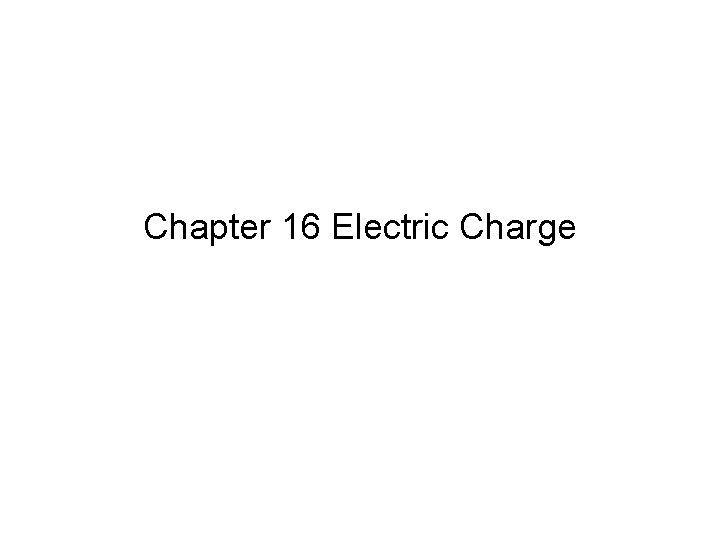Chapter 16 Electric ChargeElectric charges of the same sign A. attract each other. B. repel each other. C. exert no forces on each other.Electric charges of the same sign A. attract each other. B. repel each other. C. exert no forces on each other.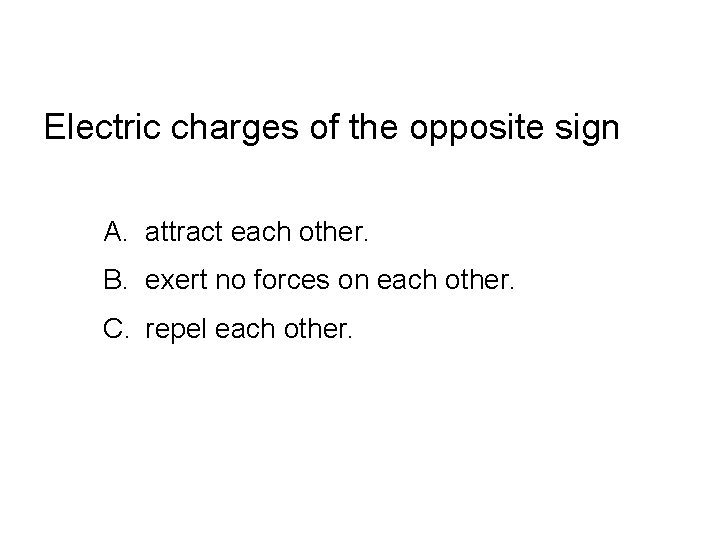Electric charges of the opposite sign A. attract each other. B. exert no forces on each other. C. repel each other.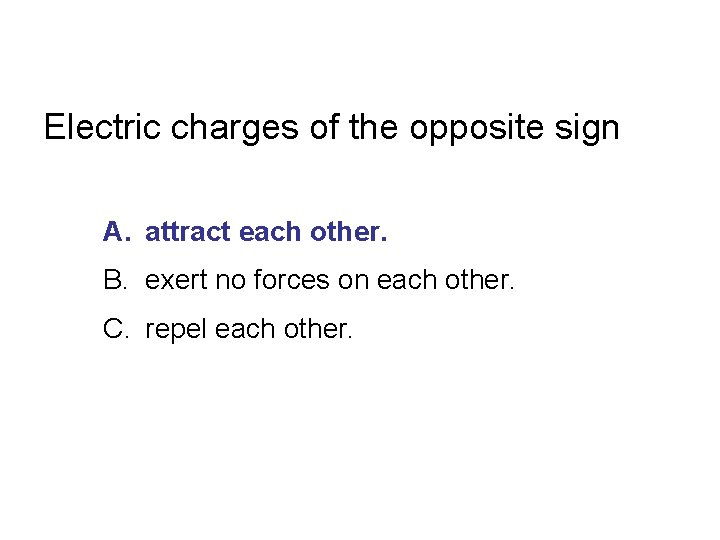Electric charges of the opposite sign A. attract each other. B. exert no forces on each other. C. repel each other.Experimental evidence indicates that A. charge is quantized and conserved. B. charge is quantized but not conserved. C. charge is conserved but not quantized. D. charge is neither quantized nor conserved.Experimental evidence indicates that A. charge is quantized and conserved. B. charge is quantized but not conserved. C. charge is conserved but not quantized. D. charge is neither quantized nor conserved.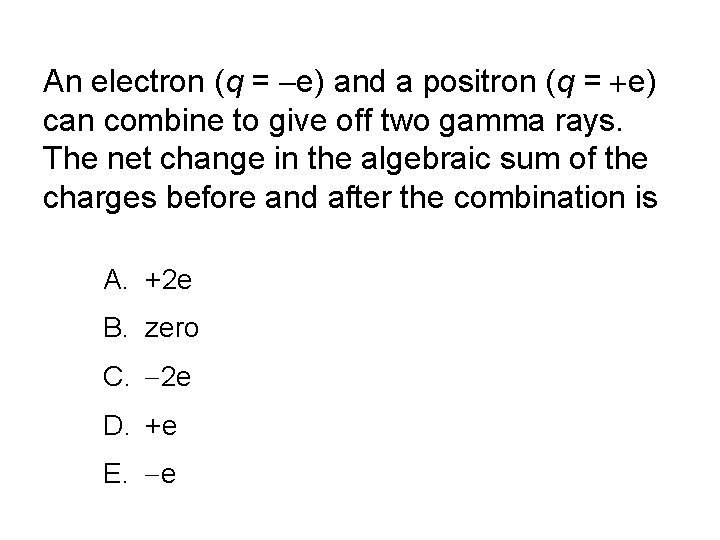An electron (q = e) and a positron (q = e) can combine to give off two gamma rays. The net change in the algebraic sum of the charges before and after the combination is A. +2 e B. zero C. 2 e D. +e E. e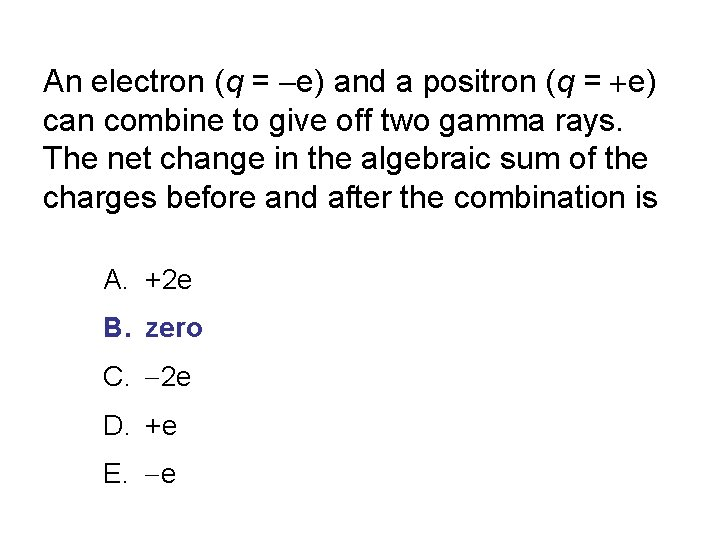An electron (q = e) and a positron (q = e) can combine to give off two gamma rays. The net change in the algebraic sum of the charges before and after the combination is A. +2 e B. zero C. 2 e D. +e E. e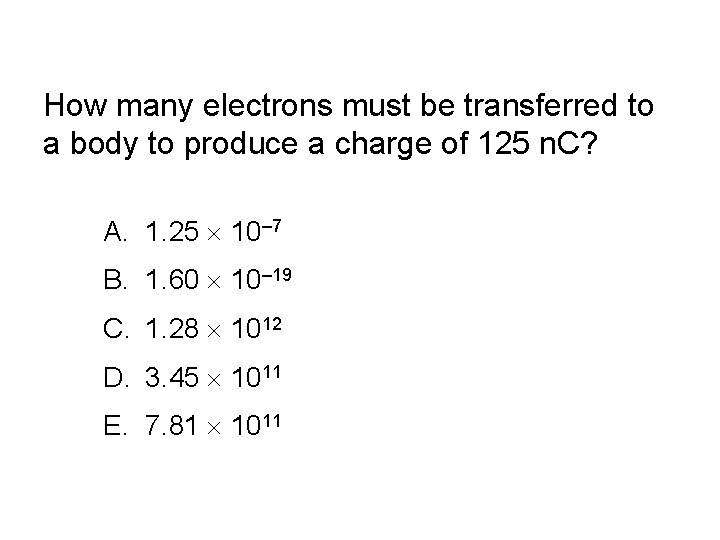How many electrons must be transferred to a body to produce a charge of 125 n. C? A. 1. 25 10– 7 B. 1. 60 10– 19 C. 1. 28 1012 D. 3. 45 1011 E. 7. 81 1011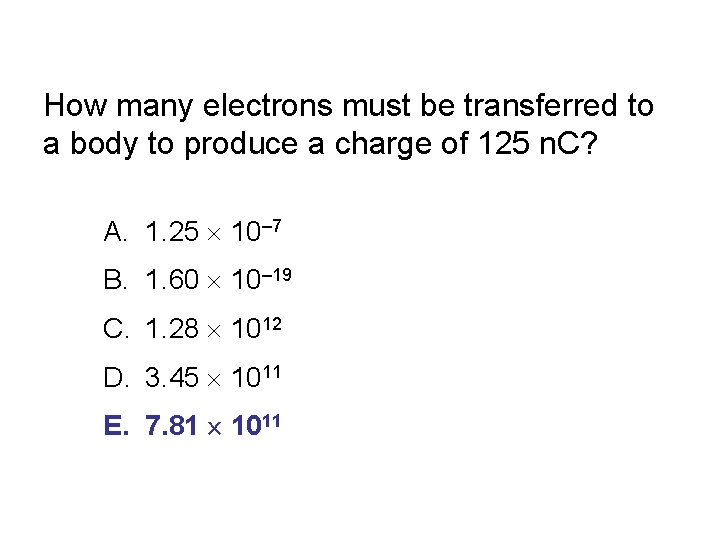How many electrons must be transferred to a body to produce a charge of 125 n. C? A. 1. 25 10– 7 B. 1. 60 10– 19 C. 1. 28 1012 D. 3. 45 1011 E. 7. 81 1011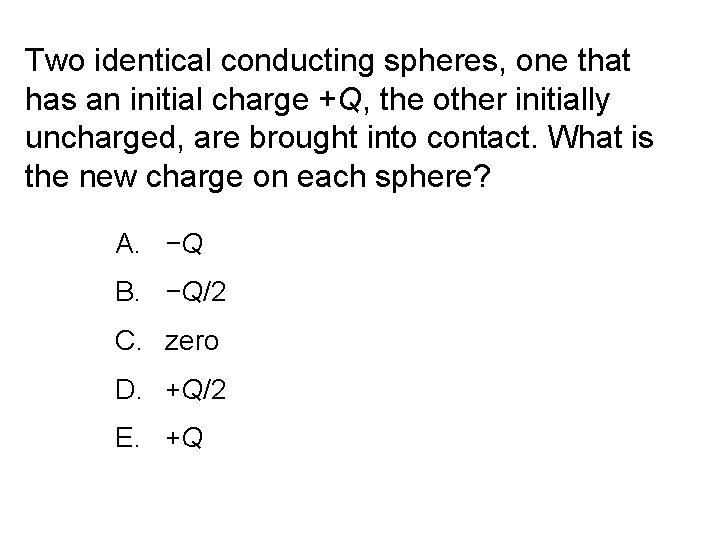Two identical conducting spheres, one that has an initial charge +Q, the other initially uncharged, are brought into contact. What is the new charge on each sphere? A. −Q B. −Q/2 C. zero D. +Q/2 E. +QTwo identical conducting spheres, one that has an initial charge +Q, the other initially uncharged, are brought into contact. What is the new charge on each sphere? A. −Q B. −Q/2 C. zero D. +Q/2 E. +QTwo identical conducting spheres, one that has an initial charge +Q, the other initially uncharged, are brought into contact. While the spheres are in contact, a positively charged rod is moved close to one sphere, causing a redistribution of the charges on the two spheres so the charge on the sphere closest to the rod has a charge of −Q. What is the charge on the other sphere? A. − 2 Q B. −Q C. zero D. +Q E. +2 QTwo identical conducting spheres, one that has an initial charge +Q, the other initially uncharged, are brought into contact. While the spheres are in contact, a positively charged rod is moved close to one sphere, causing a redistribution of the charges on the two spheres so the charge on the sphere closest to the rod has a charge of −Q. What is the charge on the other sphere? A. − 2 Q B. −Q C. zero D. +Q E. +2 Q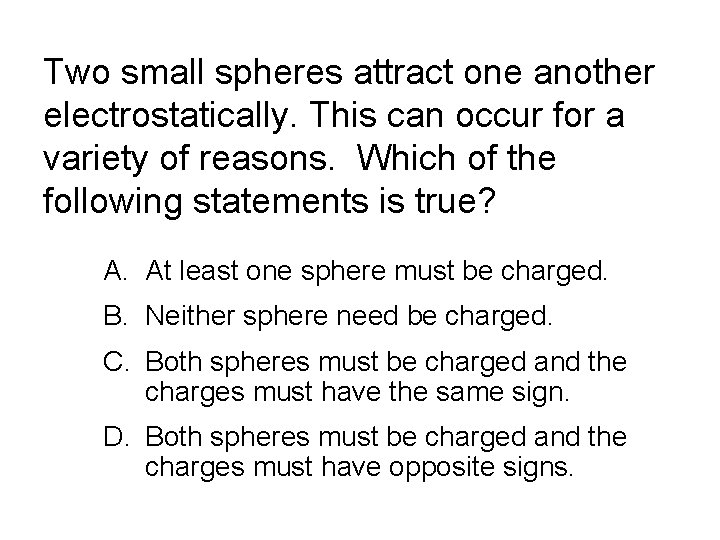Two small spheres attract one another electrostatically. This can occur for a variety of reasons. Which of the following statements is true? A. At least one sphere must be charged. B. Neither sphere need be charged. C. Both spheres must be charged and the charges must have the same sign. D. Both spheres must be charged and the charges must have opposite signs.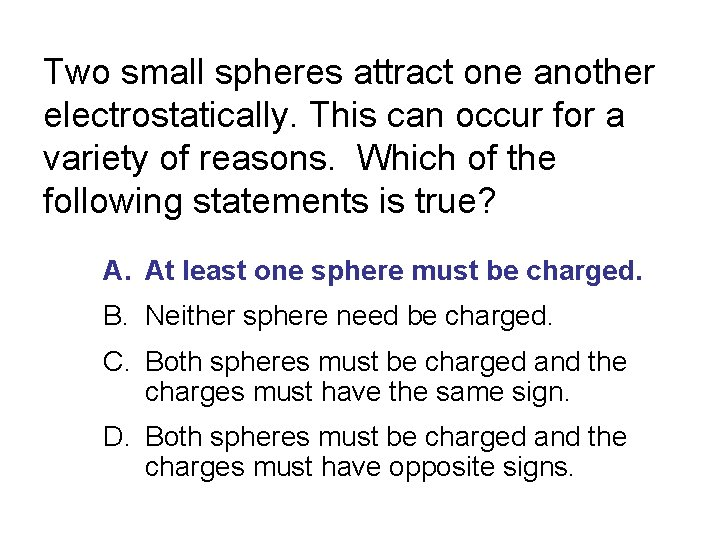Two small spheres attract one another electrostatically. This can occur for a variety of reasons. Which of the following statements is true? A. At least one sphere must be charged. B. Neither sphere need be charged. C. Both spheres must be charged and the charges must have the same sign. D. Both spheres must be charged and the charges must have opposite signs.Two small spheres repel one another electrostatically. Which of the following statements is true? A. At least one sphere must be charged. B. Neither sphere need be charged. C. Both spheres must be charged and the charges must have the same sign. D. Both spheres must be charged and the charges must have opposite signs.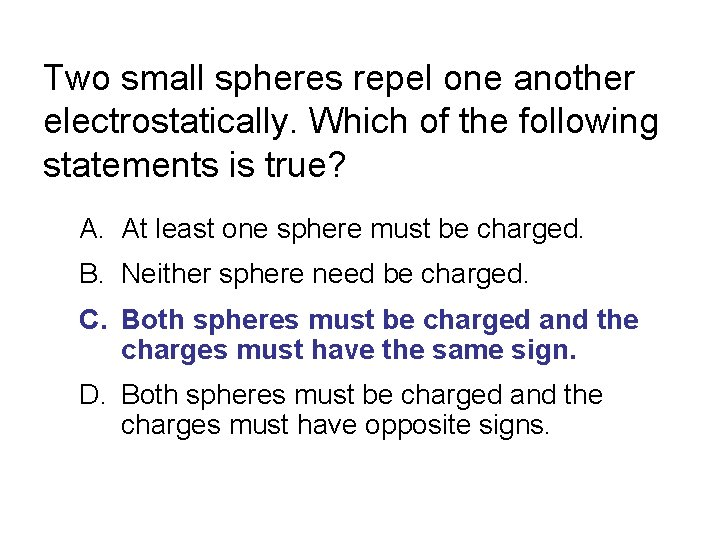Two small spheres repel one another electrostatically. Which of the following statements is true? A. At least one sphere must be charged. B. Neither sphere need be charged. C. Both spheres must be charged and the charges must have the same sign. D. Both spheres must be charged and the charges must have opposite signs.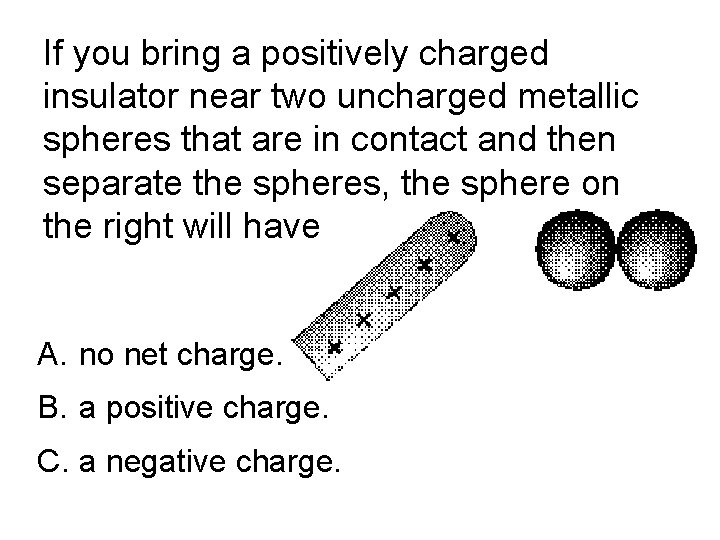If you bring a positively charged insulator near two uncharged metallic spheres that are in contact and then separate the spheres, the sphere on the right will have A. no net charge. B. a positive charge. C. a negative charge.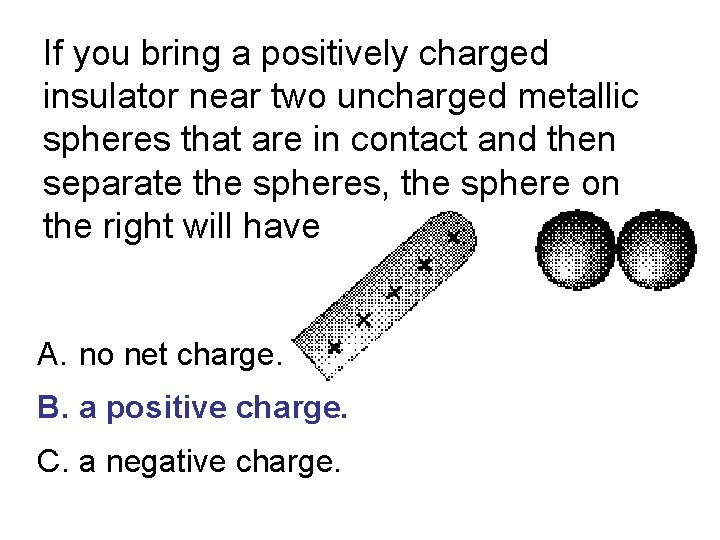If you bring a positively charged insulator near two uncharged metallic spheres that are in contact and then separate the spheres, the sphere on the right will have A. no net charge. B. a positive charge. C. a negative charge.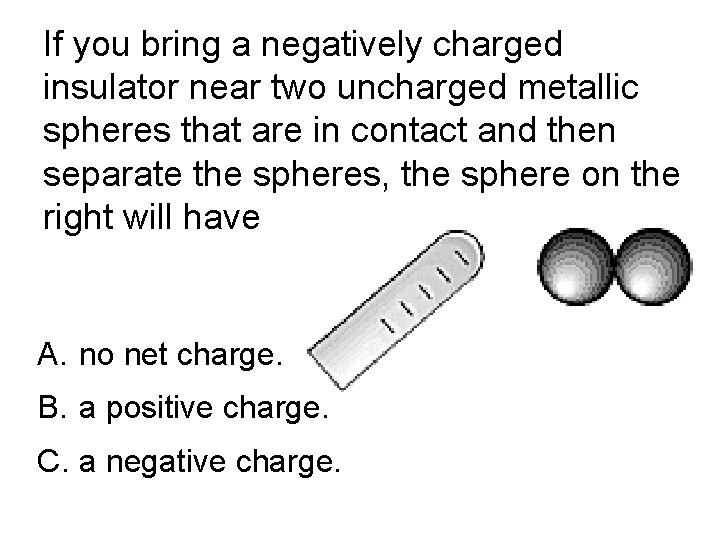If you bring a negatively charged insulator near two uncharged metallic spheres that are in contact and then separate the spheres, the sphere on the right will have A. no net charge. B. a positive charge. C. a negative charge.If you bring a negatively charged insulator near two uncharged metallic spheres that are in contact and then separate the spheres, the sphere on the right will have A. no net charge. B. a positive charge. C. a negative charge.Two small spheres, each with mass m = 5. 0 g and charge q, are suspended from a point by threads of length L = 0. 30 m. What is the charge on each sphere if the threads make an angle theta of 20º with respect to the vertical? A. 7. 9 × 10– 7 C B. 2. 9 × 10– 7 C C. 7. 5 × 10– 2 C D. 6. 3 × 10– 13 C E. 1. 8 × 10– 7 C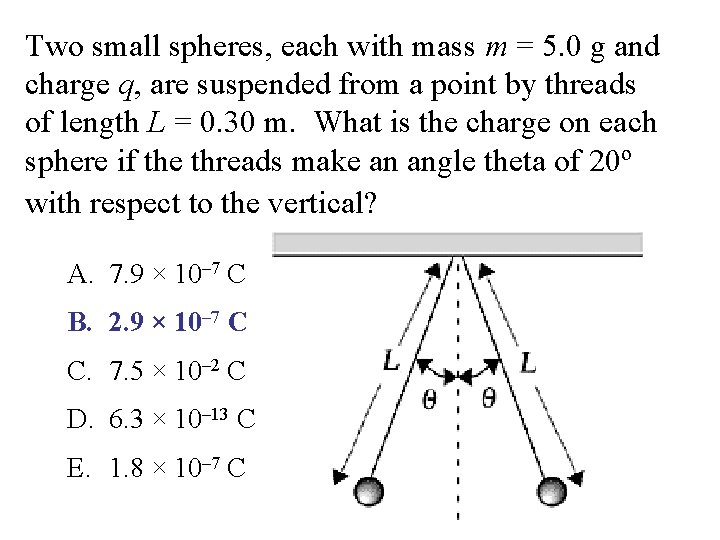Two small spheres, each with mass m = 5. 0 g and charge q, are suspended from a point by threads of length L = 0. 30 m. What is the charge on each sphere if the threads make an angle theta of 20º with respect to the vertical? A. 7. 9 × 10– 7 C B. 2. 9 × 10– 7 C C. 7. 5 × 10– 2 C D. 6. 3 × 10– 13 C E. 1. 8 × 10– 7 CThree charges +q, +Q, and –Q are placed at the corners of an equilateral triangle as shown. The net force on charge +q due to the other two charges is A. up. B. down. C. along a diagonal. D. to the left. E. to the right.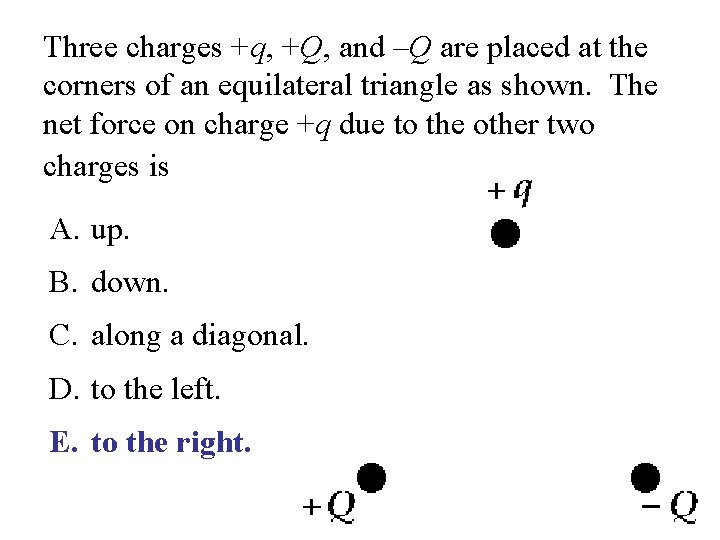Three charges +q, +Q, and –Q are placed at the corners of an equilateral triangle as shown. The net force on charge +q due to the other two charges is A. up. B. down. C. along a diagonal. D. to the left. E. to the right.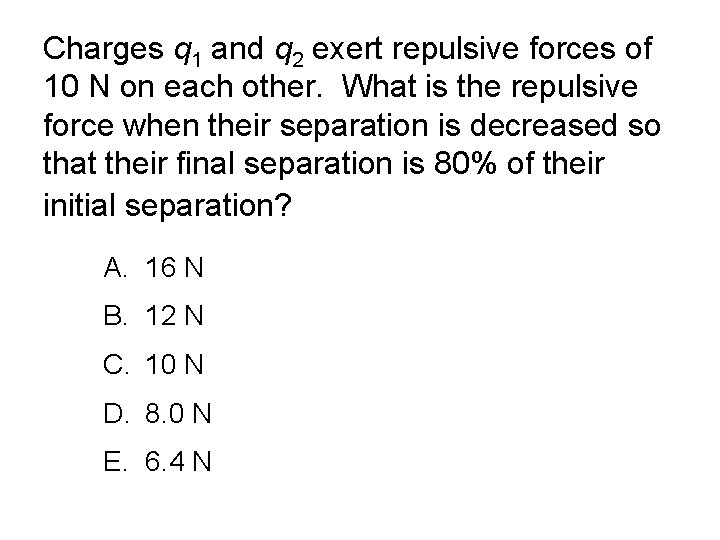Charges q 1 and q 2 exert repulsive forces of 10 N on each other. What is the repulsive force when their separation is decreased so that their final separation is 80% of their initial separation? A. 16 N B. 12 N C. 10 N D. 8. 0 N E. 6. 4 NCharges q 1 and q 2 exert repulsive forces of 10 N on each other. What is the repulsive force when their separation is decreased so that their final separation is 80% of their initial separation? A. 16 N B. 12 N C. 10 N D. 8. 0 N E. 6. 4 N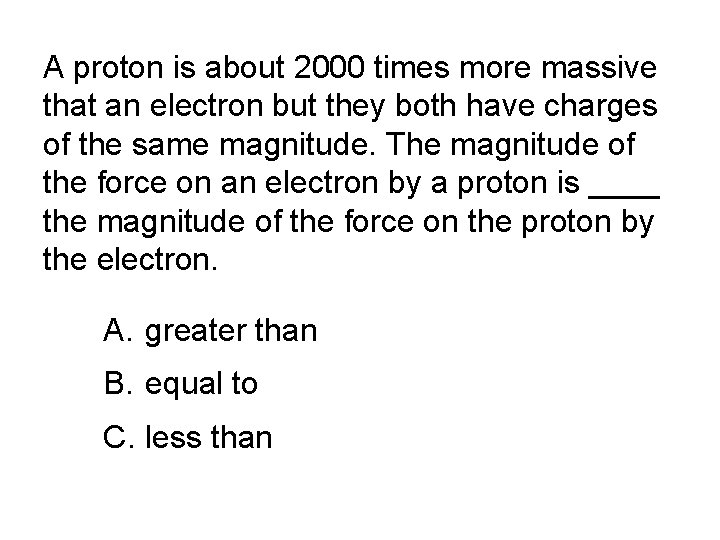A proton is about 2000 times more massive that an electron but they both have charges of the same magnitude. The magnitude of the force on an electron by a proton is ____ the magnitude of the force on the proton by the electron. A. greater than B. equal to C. less thanA proton is about 2000 times more massive that an electron but they both have charges of the same magnitude. The magnitude of the force on an electron by a proton is ____ the magnitude of the force on the proton by the electron. A. greater than B. equal to C. less thanA charge 2 Q is located at the origin while a second charge Q is located at x = a. Where should a third charge be placed so that the net force on this third charge is zero? A. x < 0 B. 0 < x < a C. x > a D. x < 0 or 0 < x < a E. 0 < x < a or x > a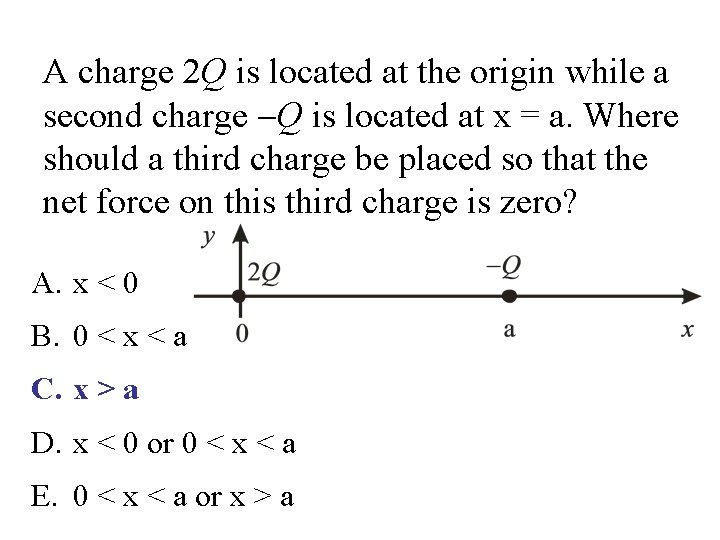A charge 2 Q is located at the origin while a second charge Q is located at x = a. Where should a third charge be placed so that the net force on this third charge is zero? A. x < 0 B. 0 < x < a C. x > a D. x < 0 or 0 < x < a E. 0 < x < a or x > aThe force between two very small charged bodies is found to be F. If the distance between them is doubled without altering their charges, the force between them becomes A. F/2 B. 2 F C. F/4 D. 4 F E. 1/F 2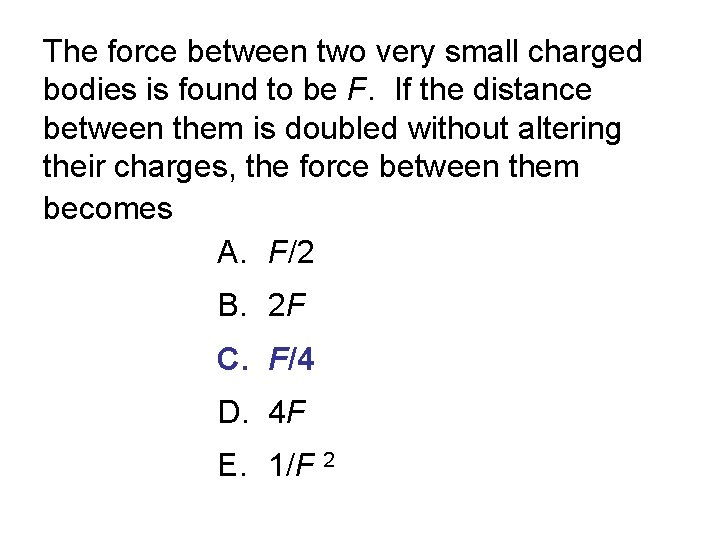The force between two very small charged bodies is found to be F. If the distance between them is doubled without altering their charges, the force between them becomes A. F/2 B. 2 F C. F/4 D. 4 F E. 1/F 2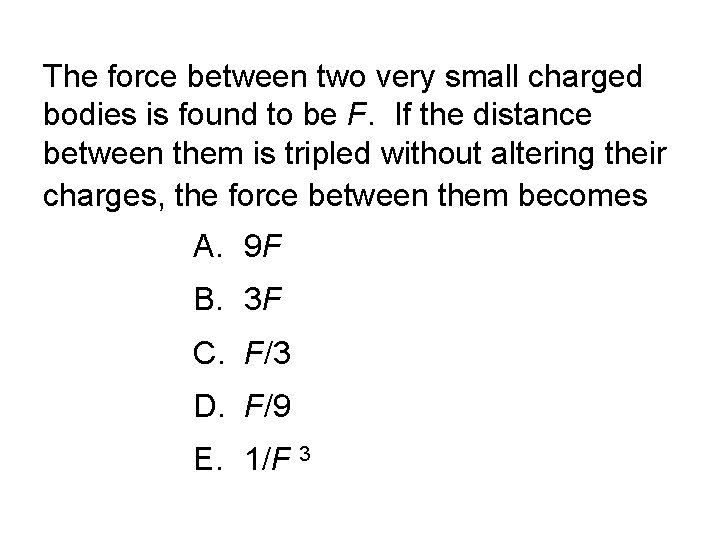The force between two very small charged bodies is found to be F. If the distance between them is tripled without altering their charges, the force between them becomes A. 9 F B. 3 F C. F/3 D. F/9 E. 1/F 3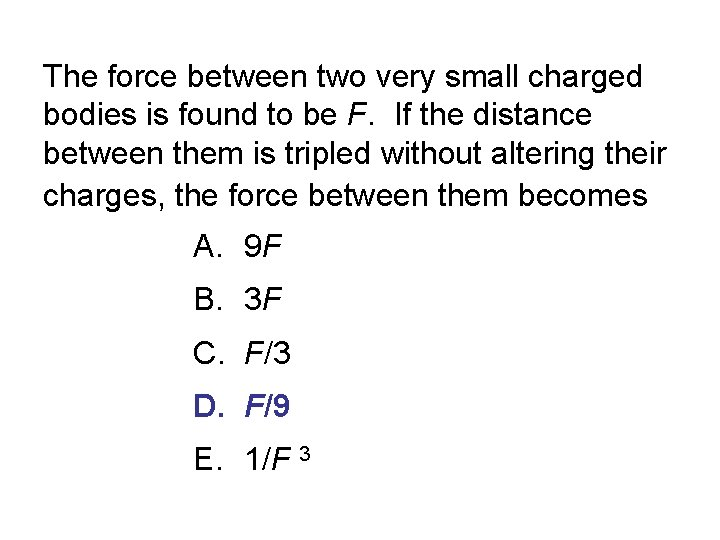The force between two very small charged bodies is found to be F. If the distance between them is tripled without altering their charges, the force between them becomes A. 9 F B. 3 F C. F/3 D. F/9 E. 1/F 3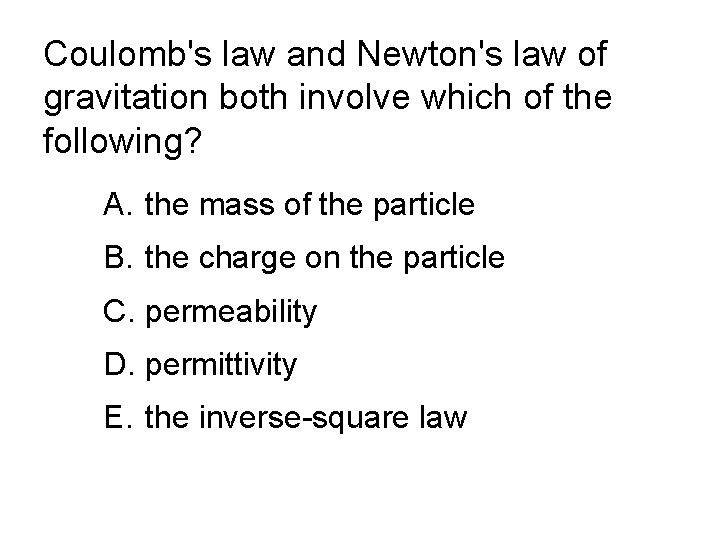Coulomb's law and Newton's law of gravitation both involve which of the following? A. the mass of the particle B. the charge on the particle C. permeability D. permittivity E. the inverse-square lawCoulomb's law and Newton's law of gravitation both involve which of the following? A. the mass of the particle B. the charge on the particle C. permeability D. permittivity E. the inverse-square law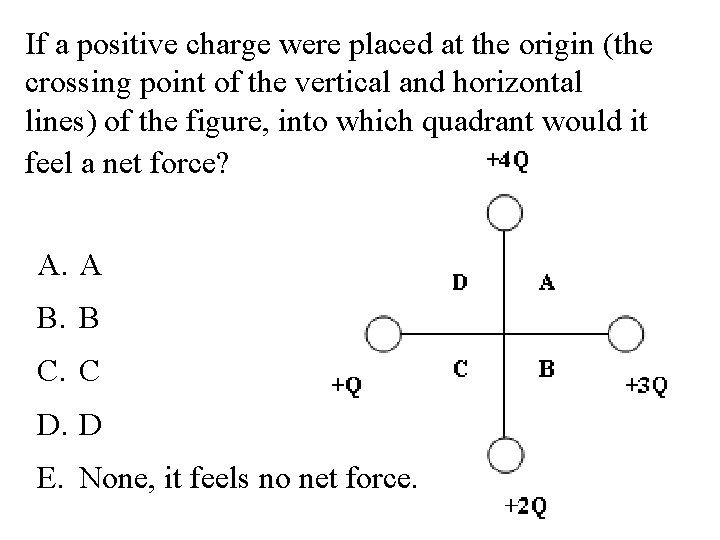If a positive charge were placed at the origin (the crossing point of the vertical and horizontal lines) of the figure, into which quadrant would it feel a net force? A. A B. B C. C D. D E. None, it feels no net force.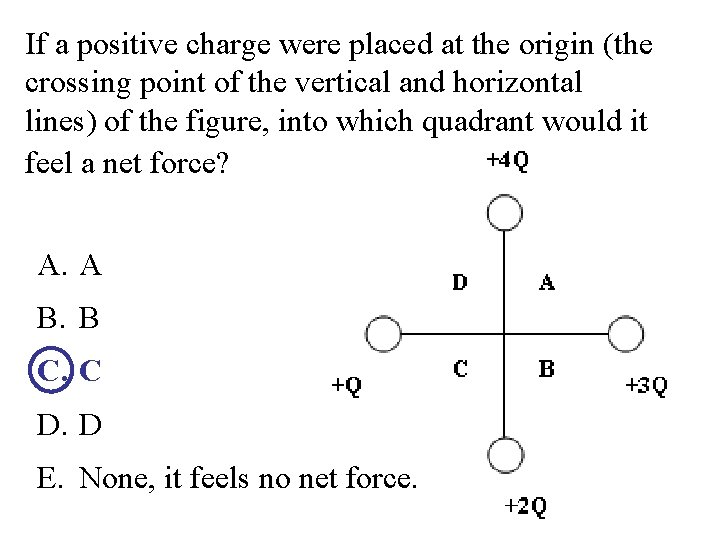If a positive charge were placed at the origin (the crossing point of the vertical and horizontal lines) of the figure, into which quadrant would it feel a net force? A. A B. B C. C D. D E. None, it feels no net force.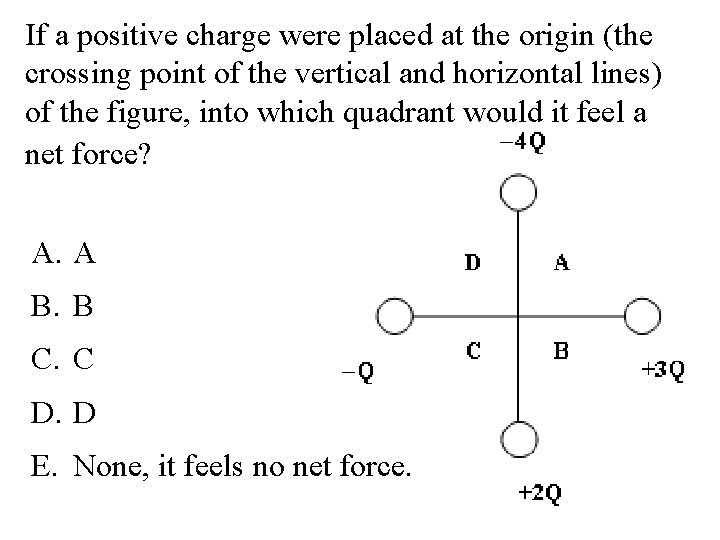If a positive charge were placed at the origin (the crossing point of the vertical and horizontal lines) of the figure, into which quadrant would it feel a net force? A. A B. B C. C D. D E. None, it feels no net force.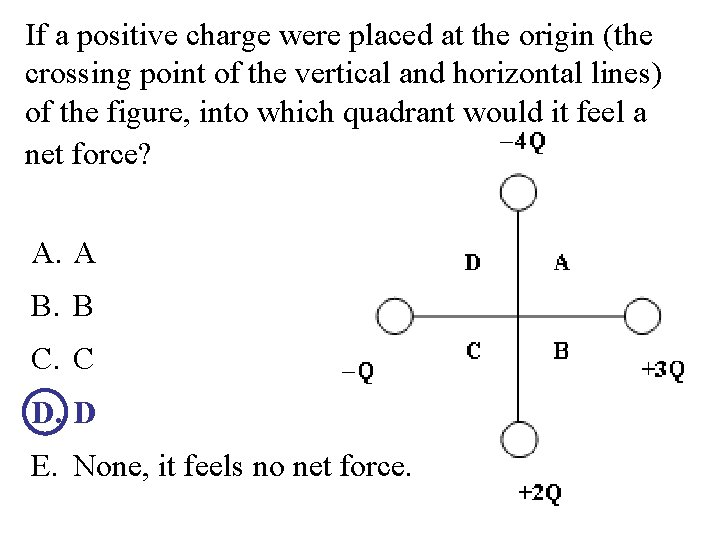If a positive charge were placed at the origin (the crossing point of the vertical and horizontal lines) of the figure, into which quadrant would it feel a net force? A. A B. B C. C D. D E. None, it feels no net force.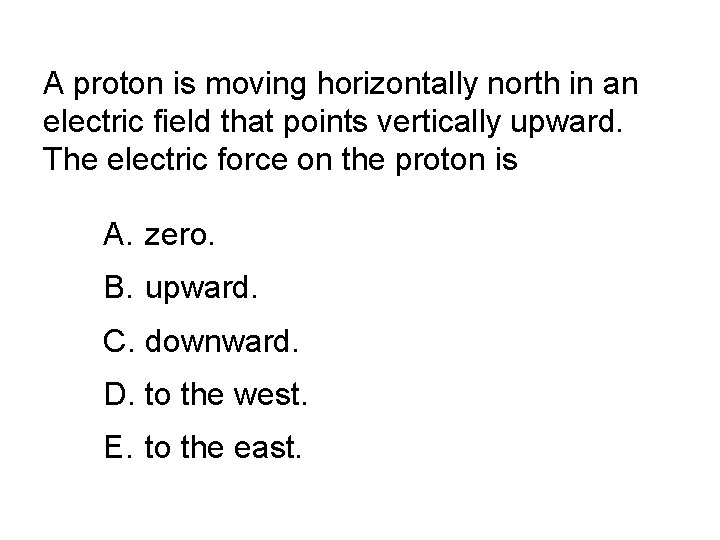A proton is moving horizontally north in an electric field that points vertically upward. The electric force on the proton is A. zero. B. upward. C. downward. D. to the west. E. to the east.A proton is moving horizontally north in an electric field that points vertically upward. The electric force on the proton is A. zero. B. upward. C. downward. D. to the west. E. to the east.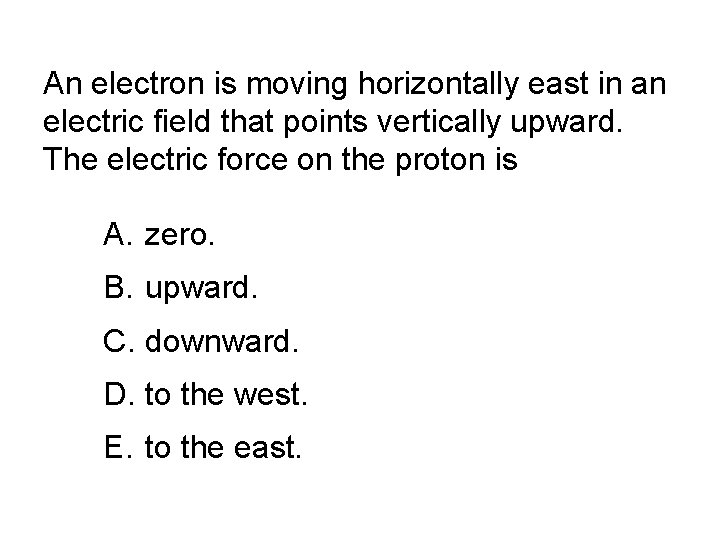An electron is moving horizontally east in an electric field that points vertically upward. The electric force on the proton is A. zero. B. upward. C. downward. D. to the west. E. to the east.An electron is moving horizontally east in an electric field that points vertically upward. The electric force on the proton is A. zero. B. upward. C. downward. D. to the west. E. to the east.Two charges of the same sign are placed a certain distance apart. There is only one point in space near them where the electric field is zero. Which, if any, of the following statements about that point is true? A. It cannot be on the line joining the charges. B. It must be on the line joining the charges and between the charges. C. It must be on the line joining the charges but not between the charges.Two charges of the same sign are placed a certain distance apart. There is only one point in space near them where the electric field is zero. Which, if any, of the following statements about that point is true? A. It cannot be on the line joining the charges. B. It must be on the line joining the charges and between the charges. C. It must be on the line joining the charges but not between the charges.Three positive and equal charges Q 1, Q 2, and Q 3 are at the corners of an equilateral triangle as shown. Point P is at the midpoint of the line between Q 1 and Q 3. The electric field at P is A. zero. B. not zero and is directed along the line from P to Q 3. C. not zero and is directed along the line from P to Q 2. D. not zero and is directed along the line from Q 1 to Q 2. E. not zero and is directed along the line from P away from Q 2.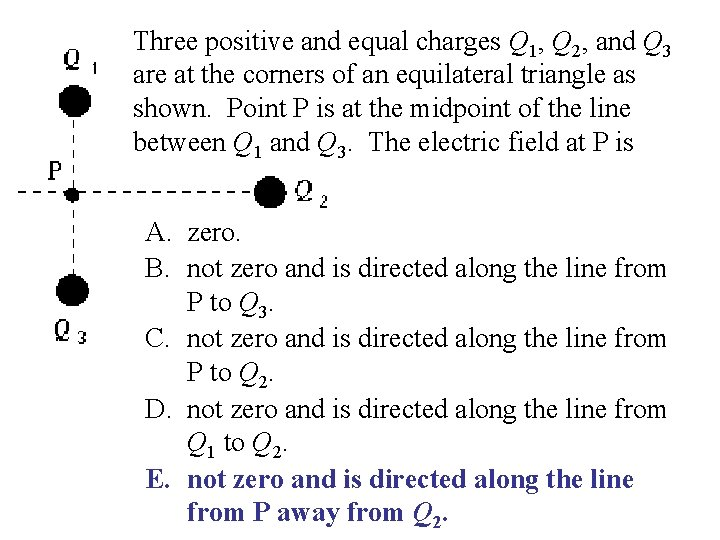Three positive and equal charges Q 1, Q 2, and Q 3 are at the corners of an equilateral triangle as shown. Point P is at the midpoint of the line between Q 1 and Q 3. The electric field at P is A. zero. B. not zero and is directed along the line from P to Q 3. C. not zero and is directed along the line from P to Q 2. D. not zero and is directed along the line from Q 1 to Q 2. E. not zero and is directed along the line from P away from Q 2.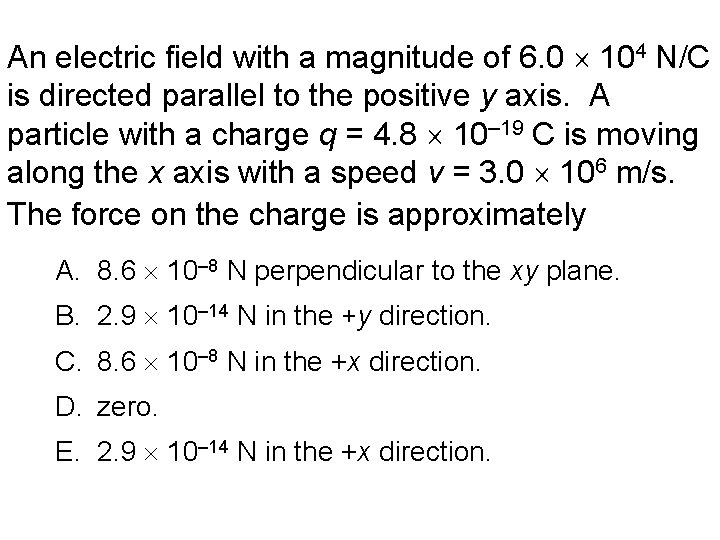An electric field with a magnitude of 6. 0 104 N/C is directed parallel to the positive y axis. A particle with a charge q = 4. 8 10– 19 C is moving along the x axis with a speed v = 3. 0 106 m/s. The force on the charge is approximately A. 8. 6 10– 8 N perpendicular to the xy plane. B. 2. 9 10– 14 N in the +y direction. C. 8. 6 10– 8 N in the +x direction. D. zero. E. 2. 9 10– 14 N in the +x direction.An electric field with a magnitude of 6. 0 104 N/C is directed parallel to the positive y axis. A particle with a charge q = 4. 8 10– 19 C is moving along the x axis with a speed v = 3. 0 106 m/s. The force on the charge is approximately A. 8. 6 10– 8 N perpendicular to the xy plane. B. 2. 9 10– 14 N in the +y direction. C. 8. 6 10– 8 N in the +x direction. D. zero. E. 2. 9 10– 14 N in the +x direction.The direction of the electric field at a point is the same as A. the direction of the force on a neutron placed at that point. B. the direction of the force on a proton placed at that point. C. the direction of the force on an electron placed at that point. D. the direction of the force on a hydrogen molecule placed at that point. E. None of these is correct.The direction of the electric field at a point is the same as A. the direction of the force on a neutron placed at that point. B. the direction of the force on a proton placed at that point. C. the direction of the force on an electron placed at that point. D. the direction of the force on a hydrogen molecule placed at that point. E. None of these is correct.Two point charges of unknown magnitude and sign are a distance d apart. If the electric field strength is zero at a point between them on the line joining them, you can conclude that A. the charges are equal in magnitude but opposite in sign. B. the charges are equal in magnitude and have the same sign. C. the charges are not necessarily equal in magnitude but have opposite signs. D. the charges are not necessarily equal in magnitude but have the same sign. E. there is not enough information to say anything specific about the charges.Two point charges of unknown magnitude and sign are a distance d apart. If the electric field strength is zero at a point between them on the line joining them, you can conclude that A. the charges are equal in magnitude but opposite in sign. B. the charges are equal in magnitude and have the same sign. C. the charges are not necessarily equal in magnitude but have opposite signs. D. the charges are not necessarily equal in magnitude but have the same sign. E. there is not enough information to say anything specific about the charges.Charges Q 1 = –q and Q 2 = +4 q are placed as shown. At which of the five positions indicated by the lettered dots might the electric field be zero?Charges Q 1 = –q and Q 2 = +4 q are placed as shown. At which of the five positions indicated by the lettered dots might the electric field be zero?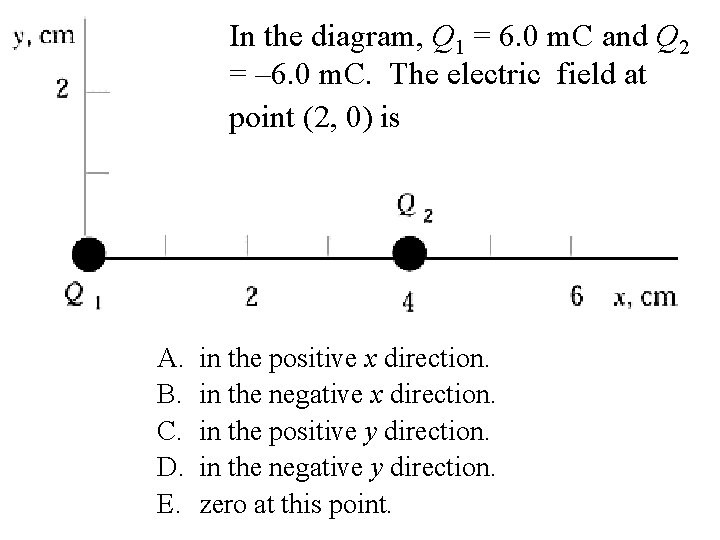In the diagram, Q 1 = 6. 0 m. C and Q 2 = – 6. 0 m. C. The electric field at point (2, 0) is A. B. C. D. E. in the positive x direction. in the negative x direction. in the positive y direction. in the negative y direction. zero at this point.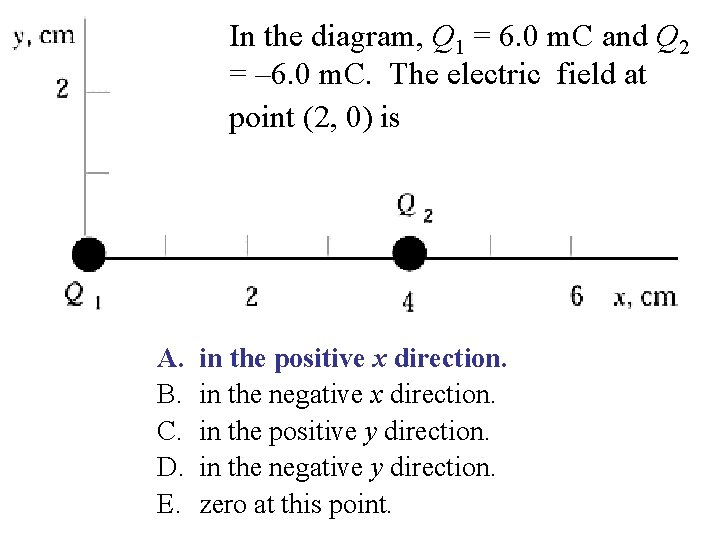In the diagram, Q 1 = 6. 0 m. C and Q 2 = – 6. 0 m. C. The electric field at point (2, 0) is A. B. C. D. E. in the positive x direction. in the negative x direction. in the positive y direction. in the negative y direction. zero at this point.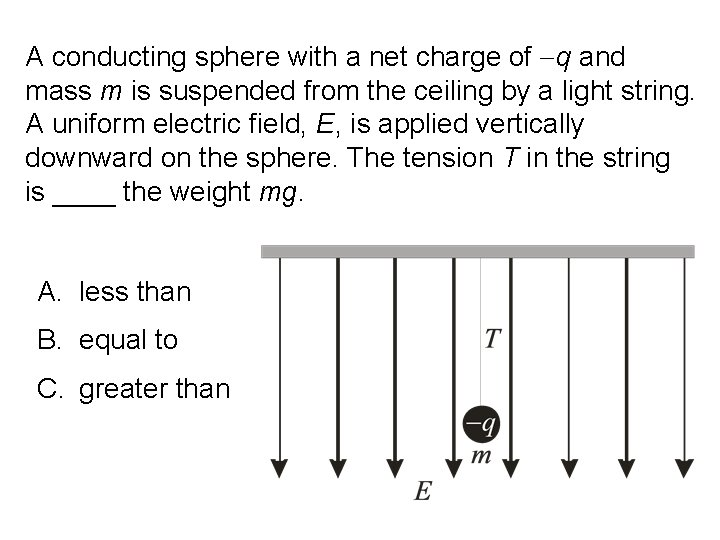A conducting sphere with a net charge of q and mass m is suspended from the ceiling by a light string. A uniform electric field, E, is applied vertically downward on the sphere. The tension T in the string is ____ the weight mg. A. less than B. equal to C. greater than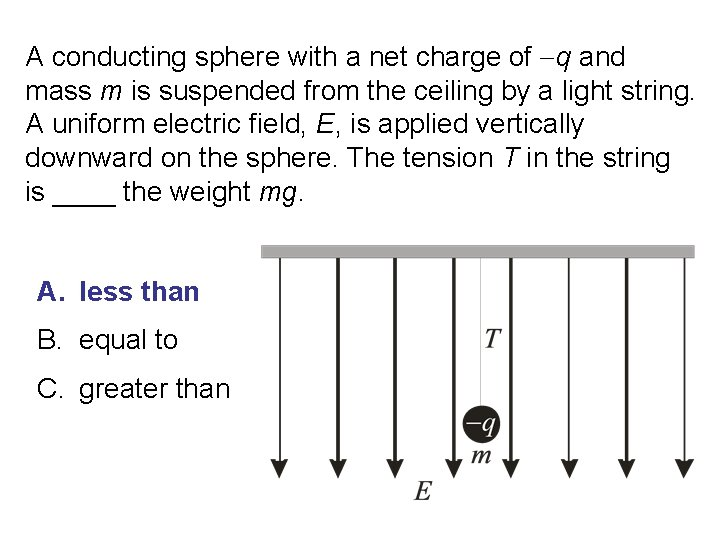A conducting sphere with a net charge of q and mass m is suspended from the ceiling by a light string. A uniform electric field, E, is applied vertically downward on the sphere. The tension T in the string is ____ the weight mg. A. less than B. equal to C. greater thanA conducting sphere with a net charge of q=− 1 C and mass m = 1 g is suspended from the ceiling by a light string. A uniform electric field, E = 5000 N/C, is applied vertically downward on the sphere. The tension T in the string is A. 5 10− 3 N B. 9. 81 10− 3 N C. 4. 81 10− 3 N D. 1. 48 10− 2 N E. zero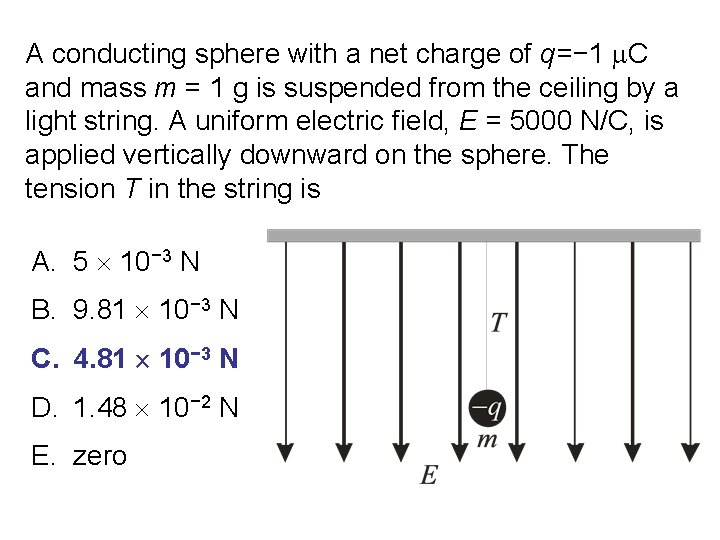A conducting sphere with a net charge of q=− 1 C and mass m = 1 g is suspended from the ceiling by a light string. A uniform electric field, E = 5000 N/C, is applied vertically downward on the sphere. The tension T in the string is A. 5 10− 3 N B. 9. 81 10− 3 N C. 4. 81 10− 3 N D. 1. 48 10− 2 N E. zero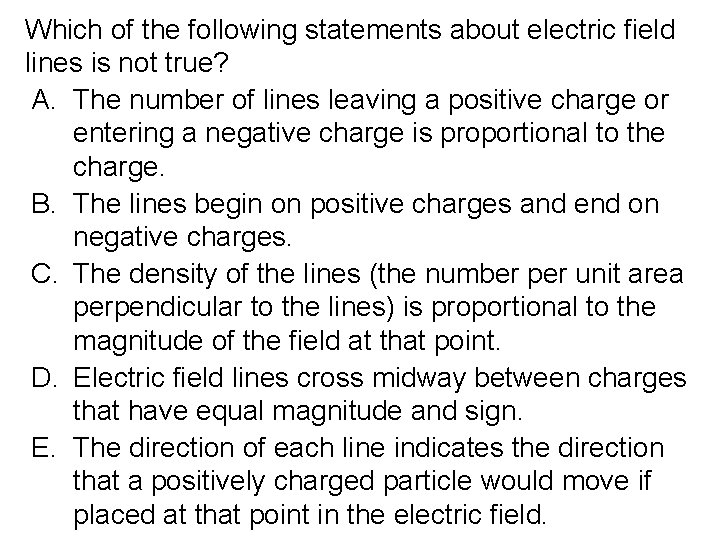Which of the following statements about electric field lines is not true? A. The number of lines leaving a positive charge or entering a negative charge is proportional to the charge. B. The lines begin on positive charges and end on negative charges. C. The density of the lines (the number per unit area perpendicular to the lines) is proportional to the magnitude of the field at that point. D. Electric field lines cross midway between charges that have equal magnitude and sign. E. The direction of each line indicates the direction that a positively charged particle would move if placed at that point in the electric field.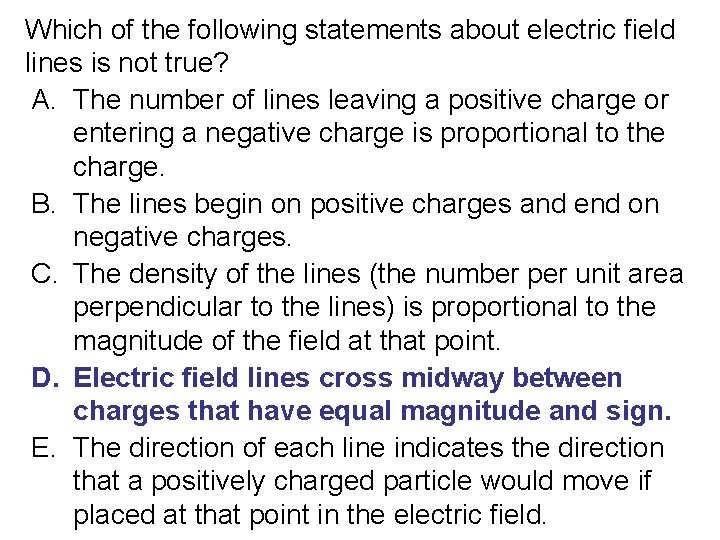Which of the following statements about electric field lines is not true? A. The number of lines leaving a positive charge or entering a negative charge is proportional to the charge. B. The lines begin on positive charges and end on negative charges. C. The density of the lines (the number per unit area perpendicular to the lines) is proportional to the magnitude of the field at that point. D. Electric field lines cross midway between charges that have equal magnitude and sign. E. The direction of each line indicates the direction that a positively charged particle would move if placed at that point in the electric field.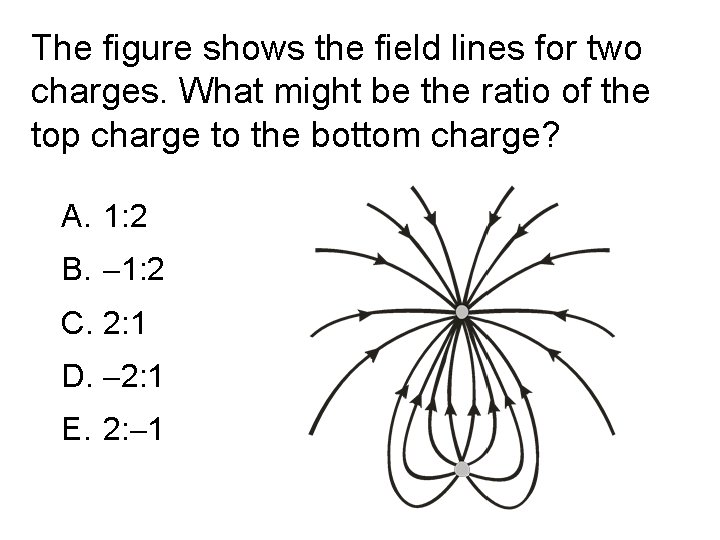The figure shows the field lines for two charges. What might be the ratio of the top charge to the bottom charge? A. 1: 2 B. 1: 2 C. 2: 1 D. 2: 1 E. 2: 1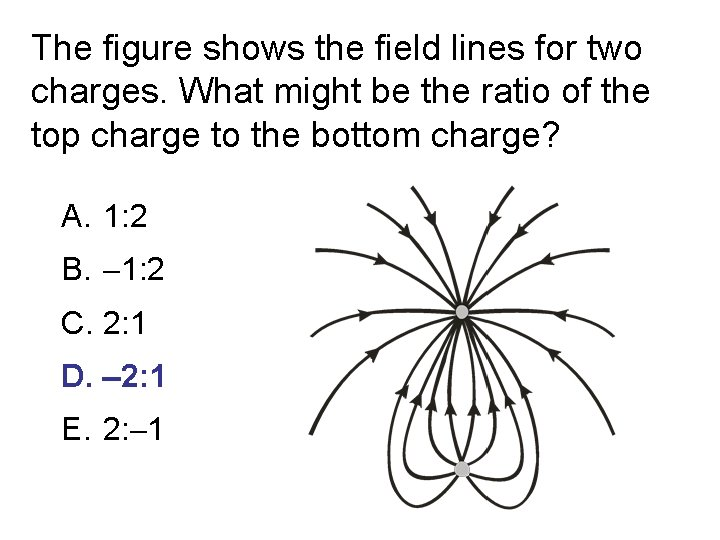The figure shows the field lines for two charges. What might be the ratio of the top charge to the bottom charge? A. 1: 2 B. 1: 2 C. 2: 1 D. 2: 1 E. 2: 1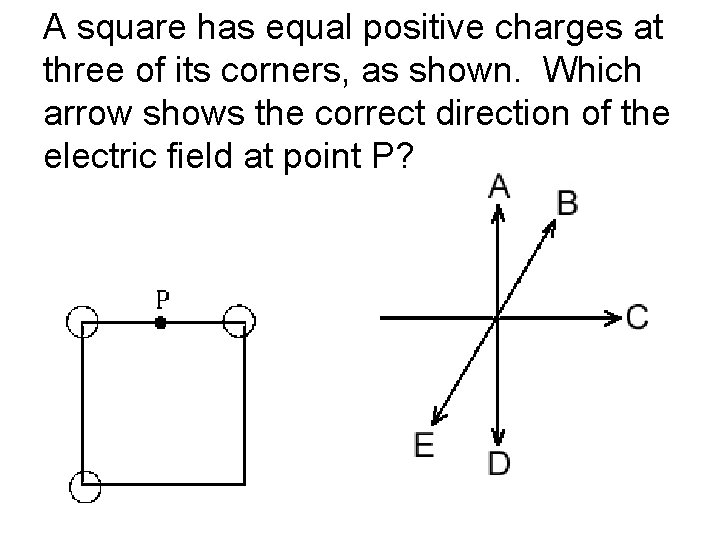A square has equal positive charges at three of its corners, as shown. Which arrow shows the correct direction of the electric field at point P?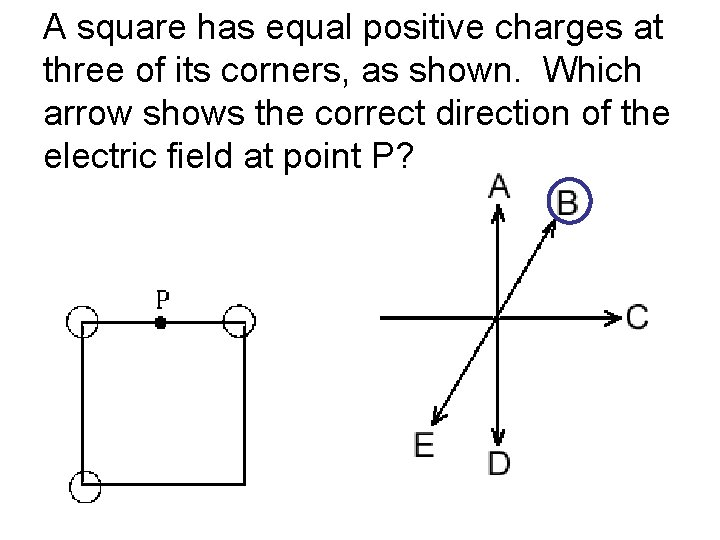A square has equal positive charges at three of its corners, as shown. Which arrow shows the correct direction of the electric field at point P?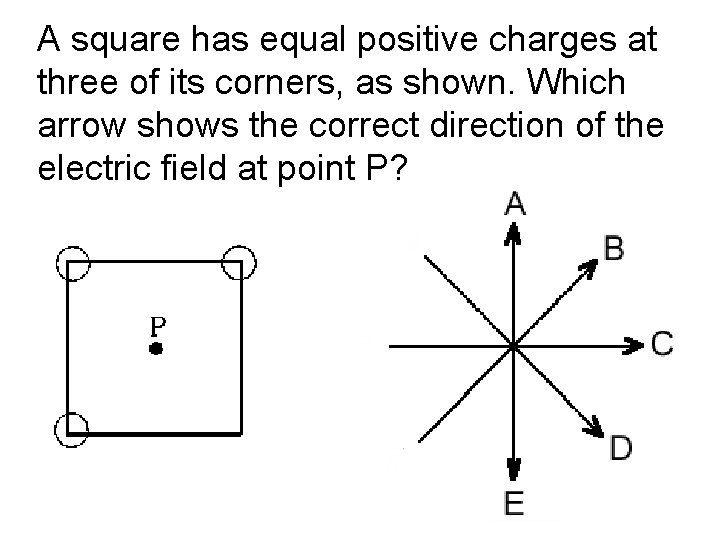A square has equal positive charges at three of its corners, as shown. Which arrow shows the correct direction of the electric field at point P?A square has equal positive charges at three of its corners, as shown. Which arrow shows the correct direction of the electric field at point P?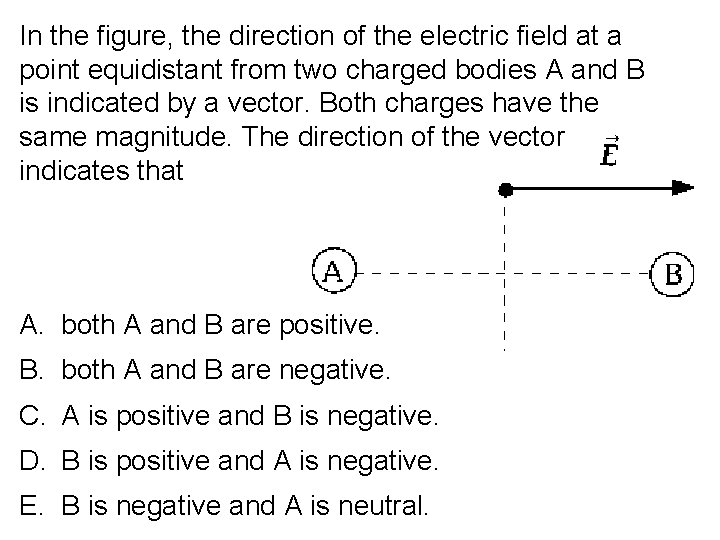In the figure, the direction of the electric field at a point equidistant from two charged bodies A and B is indicated by a vector. Both charges have the same magnitude. The direction of the vector indicates that A. both A and B are positive. B. both A and B are negative. C. A is positive and B is negative. D. B is positive and A is negative. E. B is negative and A is neutral.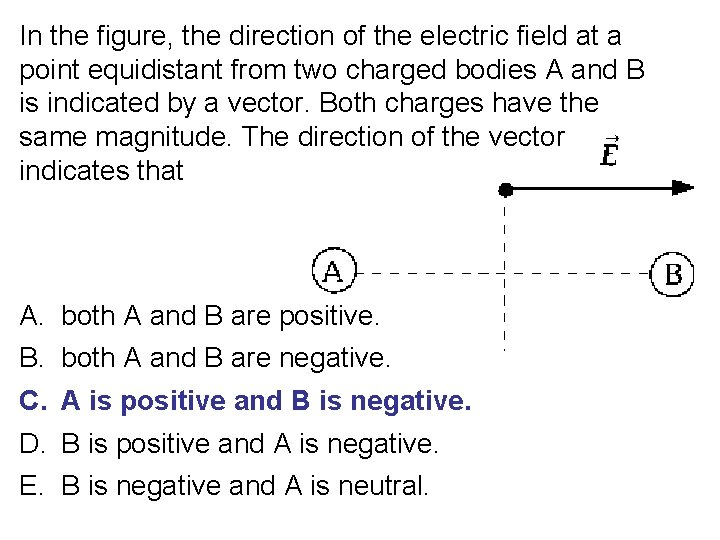In the figure, the direction of the electric field at a point equidistant from two charged bodies A and B is indicated by a vector. Both charges have the same magnitude. The direction of the vector indicates that A. B. C. D. E. both A and B are positive. both A and B are negative. A is positive and B is negative. B is positive and A is negative. B is negative and A is neutral.Chapter 21: The Electric Field I: Discrete Charge Distributions Section 21 -6: Action of the Electric Field on ChargesIf nonelectric forces are negligible, in a uniform electric field a proton has A. a constant velocity in the direction of the field. B. a constant velocity in a direction opposite to that of the field. C. a constant acceleration the direction of the field. D. a constant acceleration in a direction opposite to that of the field. E. a constant acceleration in a direction at right angles to the field.If nonelectric forces are negligible, in a uniform electric field a proton has A. a constant velocity in the direction of the field. B. a constant velocity in a direction opposite to that of the field. C. a constant acceleration the direction of the field. D. a constant acceleration in a direction opposite to that of the field. E. a constant acceleration in a direction at right angles to the field.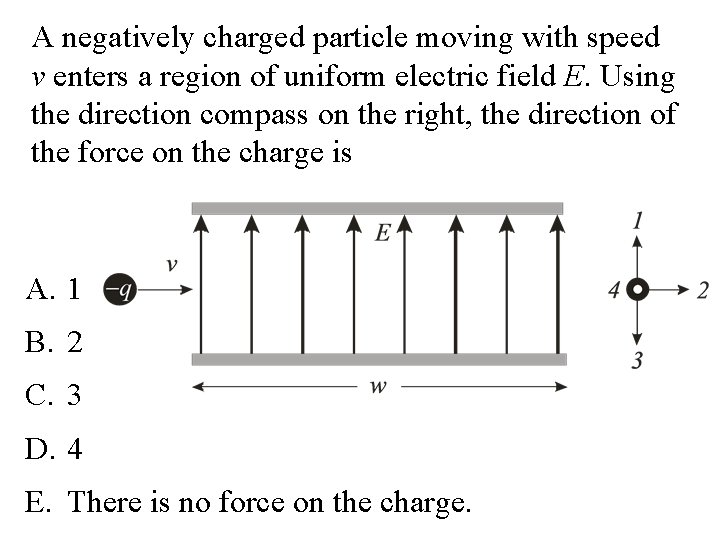A negatively charged particle moving with speed v enters a region of uniform electric field E. Using the direction compass on the right, the direction of the force on the charge is A. 1 B. 2 C. 3 D. 4 E. There is no force on the charge.A negatively charged particle moving with speed v enters a region of uniform electric field E. Using the direction compass on the right, the direction of the force on the charge is A. 1 B. 2 C. 3 D. 4 E. There is no force on the charge.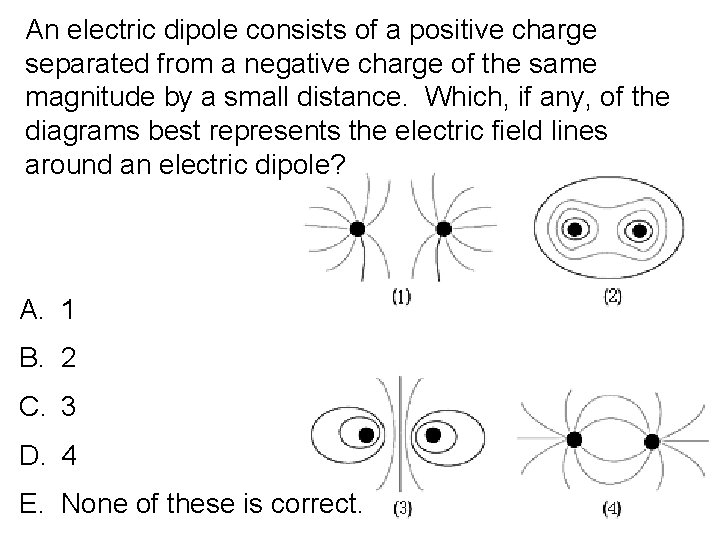An electric dipole consists of a positive charge separated from a negative charge of the same magnitude by a small distance. Which, if any, of the diagrams best represents the electric field lines around an electric dipole? A. 1 B. 2 C. 3 D. 4 E. None of these is correct.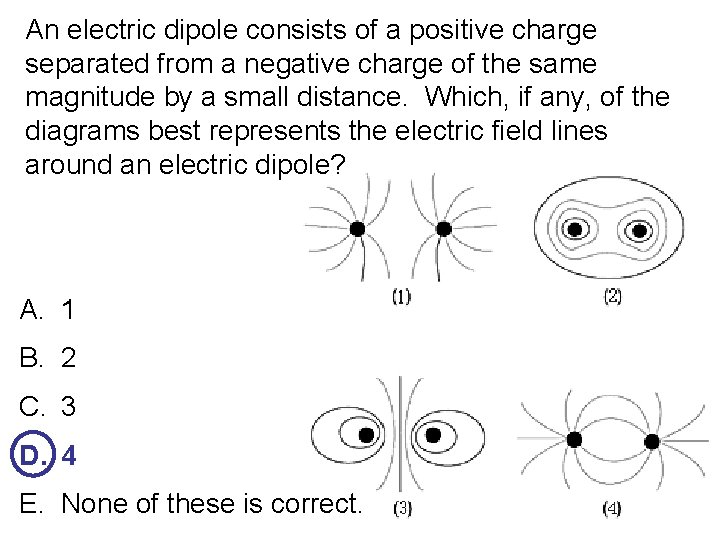An electric dipole consists of a positive charge separated from a negative charge of the same magnitude by a small distance. Which, if any, of the diagrams best represents the electric field lines around an electric dipole? A. 1 B. 2 C. 3 D. 4 E. None of these is correct.